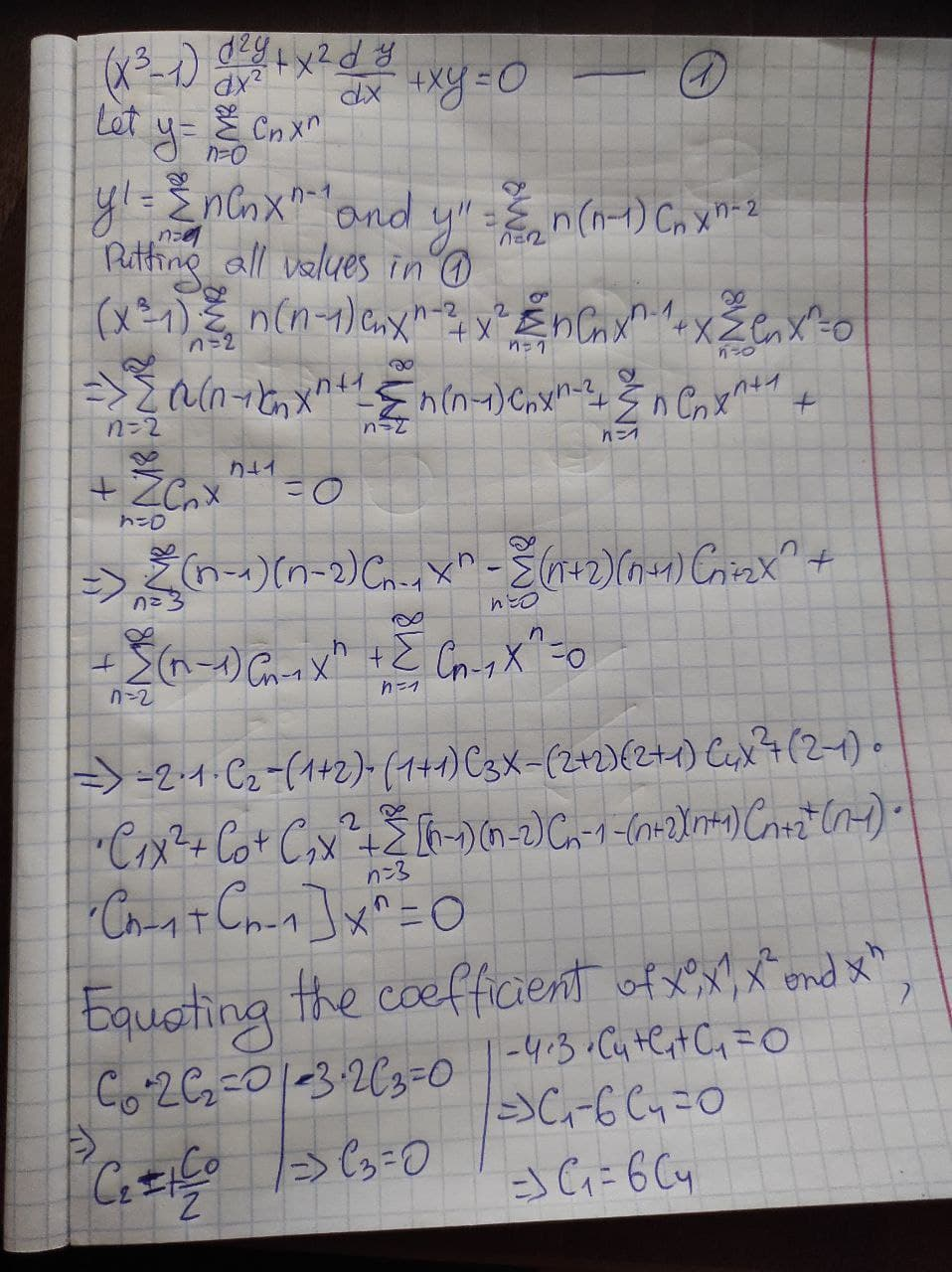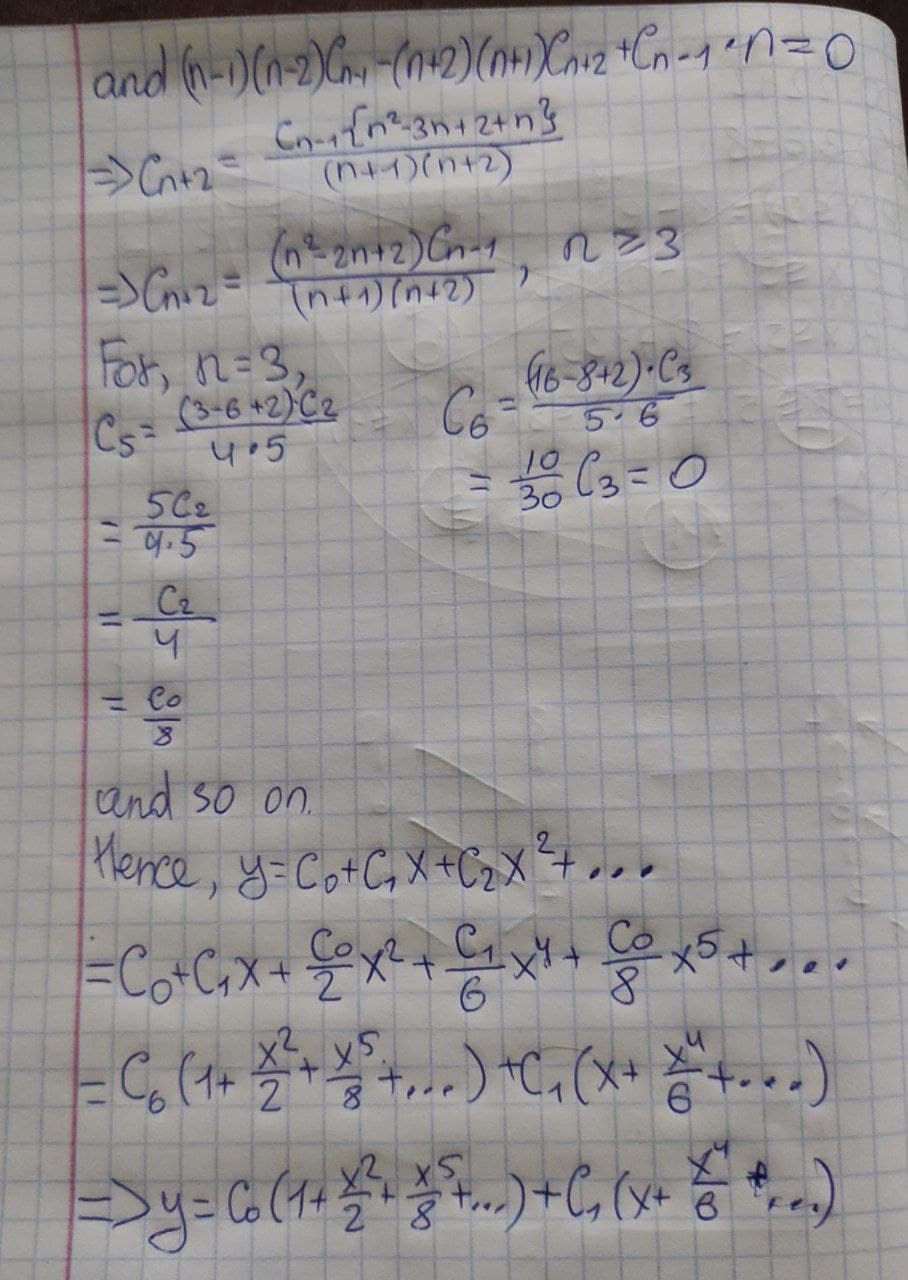# Find the power series solution of the differentialghostmasakii 2022-01-28 Answered

Find the power series solution of the differential equation given below around the point X0=0. $\left({x}^{3}-1\right)\left({d}^{2}y/d{x}^{2}\right)+{x}^{2}\left(dy/dx\right)+xy=0$

You can still ask an expert for help

## Want to know more about Laplace transform?

• Live experts 24/7
• Questions are typically answered in as fast as 30 minutes
• Personalized clear answers

Solve your problem for the price of one coffee

• Math expert for every subject
• Pay only if we can solve italenahelenash

Solution is on photo belowWe have step-by-step solutions for your answer!